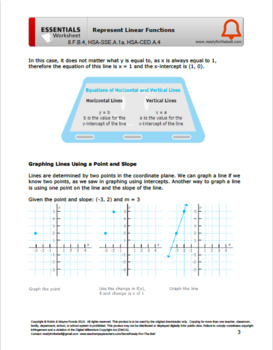# HSF.IF.A.1 8.F.B.4 Interpreting Functions: Representing Linear FunctionsSubject
Resource Type
File Type

Zip

(2 MB|11+11)
Standards
Also included in:
1. This bundle contains 29 CCSS Algebra Essentials Study Guides and 29 Answer Keys. In all, more than 400 pages to help you get Ready for the Bell.Essentials worksheets give students review, guided, and independent practice in preparation for assessment, remediation post-assessment, and as a supplement
\$84.00
\$75.60
Save \$8.40
• Product Description
• Standards

This 11+11 page Essentials worksheet and answer key focuses on representing linear equations.

- Identifying intercepts using standard form

- Graphing lines using a point and slope

- Graph using slope intercept form

Essentials worksheets give students review, guided, and independent practice in preparation for assessment, remediation post-assessment, and as a supplemental resource for RTI - response to intervention. The worksheets can be used in the classroom or as homework and study preparation. They also work well for summer school skills catch-up.

This download contains 22 pages: an 11 page worksheet, plus an easy to use 11 page answer key for the teacher or student/parent.

This Essential Worksheet and Answer Key are included at a significant discount with 28 other Study Guides with Answer Keys here:

Algebra Essentials 29 Study Guide Bundle

CC Alignment:

Functions 8.F.B.4: Use functions to model relationships between quantities.

Construct a function to model a linear relationship between two quantities. Determine the rate of change and initial value of the function from a description of a relationship or from two (x, y) values, including reading these from a table or from a graph.

HSF.IF.A.1

Understand that a function from one set (called the domain) to another set (called the range) assigns to each element of the domain exactly one element of the range. If f is a function and x is an element of its domain, then f(x) denotes the output of f corresponding to the input x. The graph of f is the graph of the equation y = f(x).

Rearrange formulas to highlight a quantity of interest, using the same reasoning as in solving equations. For example, rearrange Ohm’s law 𝘝 = 𝘭𝘙 to highlight resistance 𝘙.
Interpret parts of an expression, such as terms, factors, and coefficients.
Construct a function to model a linear relationship between two quantities. Determine the rate of change and initial value of the function from a description of a relationship or from two (𝘹, 𝘺) values, including reading these from a table or from a graph. Interpret the rate of change and initial value of a linear function in terms of the situation it models, and in terms of its graph or a table of values.
Total Pages
11+11
Included
Teaching Duration
55 minutes
Report this Resource to TpT
Reported resources will be reviewed by our team. Report this resource to let us know if this resource violates TpT’s content guidelines.